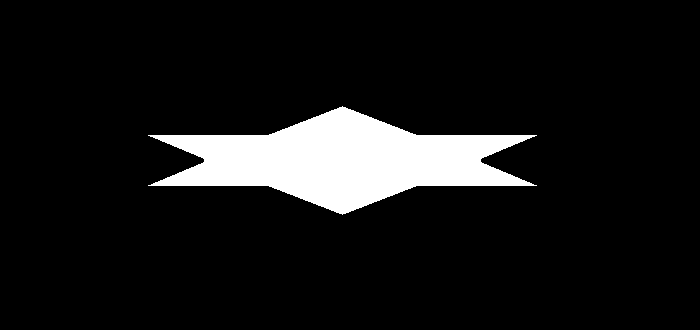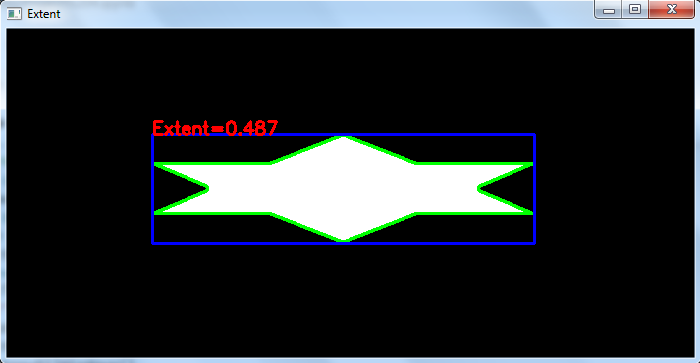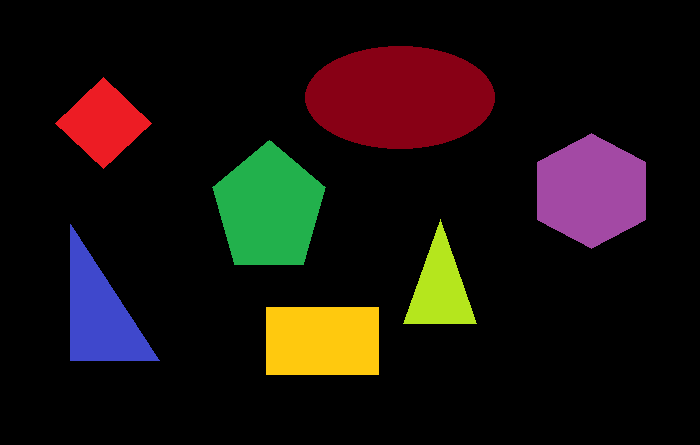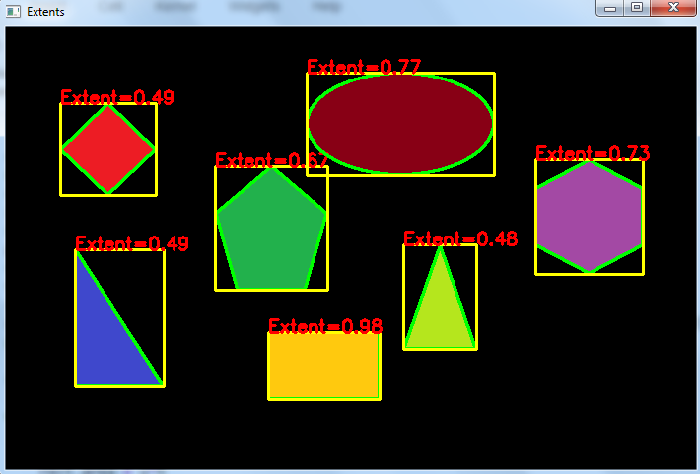# How to compute the extent of an object in image using OpenCV Python?

The extent of an object is computed as the ratio of contour area to its bounding rectangle area. So, to compute the extent, we first have to find the contour area and bounding rectangle area. The contour area of an object can be found using cv2.contourArea() function.

## Syntax

The extent can be computed as follows −

area = cv2.contourArea(cnt)
x,y,w,h = cv2.boundingRect(cnt)
rect_area = w*h
extent = float(area)/rect_area


Here, "cnt" is a numpy array of the contour points of an object in the image.

## Steps

You can use the following steps to compute extent of an object in an image −

Import the required library. In all the following Python examples, the required Python library is OpenCV. Make sure you have already installed it.

import cv2


img = cv2.imread('star1.png')
gray = cv2.cvtColor(img, cv2.COLOR_BGR2GRAY)


Apply thresholding on the grayscale image to create a binary image. Adjust the second argument for better detection of contour.

ret,thresh = cv2.threshold(gray,150,255,0)


Find the contours in the image using cv2.findContours() function.

contours, _ = cv2.findContours(thresh, cv2.RETR_TREE, cv2.CHAIN_APPROX_SIMPLE)


Select a contour "cnt" or loop over all contours. Compute the extent as the ratio of contour area to the area of bounding rectangle.

cnt = contours
area = cv2.contourArea(cnt)
x,y,w,h = cv2.boundingRect(cnt)
rect_area = w*h
extent = float(area)/rect_area


Optionally you can draw the contours and bounding rectangle on the input image. Also put the extent as text on the image

cv2.drawContours(img, [cnt], -1, (0,255,255), 3)
cv2.rectangle(img,(x,y),(x+w,y+h),(255,0,0),2)


Print the computed extent and display the image with the drawn contours, bounding rectangle.

print("Extent of the object:", ext)
cv2.imshow("Extent", img)
cv2.waitKey(0)
cv2.destroyAllWindows()


Let's have a look at some examples for a better understanding.

## Example 1

In this Python program, we compute the extent of an object in the image. We draw the contour and bounding rectangle for the object on the image. We also put the extent value as text for the object.

# import required libraries
import cv2

# convert the image to grayscale
gray = cv2.cvtColor(img, cv2.COLOR_BGR2GRAY)

# apply thresholding to convert grayscale to binary image
ret,thresh = cv2.threshold(gray,150,255,0)

# find the contours
contours,hierarchy = cv2.findContours(thresh, cv2.RETR_TREE,cv2.CHAIN_APPROX_SIMPLE)
print("Number of objects detected:", len(contours))

# define function to compute the extent
def extent(cnt):
area = cv2.contourArea(cnt)
x,y,w,h = cv2.boundingRect(cnt)
rect_area = w*h
extent = float(area)/rect_area
return extent

# select first contour
cnt = contours

# find the extent
ext = extent(cnt)

# round it to three decimal points
ext = round(ext, 3)

# draw contours
cv2.drawContours(img,[cnt],0,(0,255,0),2)

# draw bounding rectangle
x,y,w,h = cv2.boundingRect(cnt)
cv2.rectangle(img,(x,y),(x+w,y+h),(255,0,0),2)

# put text on the image
cv2.putText(img, f'Extent={ext}', (x, y), cv2.FONT_HERSHEY_SIMPLEX, 0.6, (0, 0, 255), 2)
print("Extent of the object:", ext)
cv2.imshow("Extent", img)
cv2.waitKey(0)
cv2.destroyAllWindows()


We will use the following image as the Input File in this program −## Output

When we execute the above code it will produce the following output

Number of objects detected: 1
Extent of the object:0.487


And we get the following output window −The contour is drawn in green color and the bounding rectangle in blue color. The extent of the detected object is written in red color.

## Example 2

In this Python program, we compute the extent of multiple objects in the image. We draw the contours and bounding rectangles on the image. We also put the extent as text for all objects.

# import required libraries
import cv2

# convert the image to grayscale
gray = cv2.cvtColor(img, cv2.COLOR_BGR2GRAY)

# apply thresholding to convert grayscale to binary image
ret,thresh = cv2.threshold(gray,40,255,0)

# find the contours
contours,hierarchy = cv2.findContours(thresh, cv2.RETR_TREE,cv2.CHAIN_APPROX_SIMPLE)
print("Number of objects detected:", len(contours))

# define function to compute the extent
def extent(cnt):
area = cv2.contourArea(cnt)
x,y,w,h = cv2.boundingRect(cnt)
rect_area = w*h
extent = float(area)/rect_area
return extent

# loop over all the contours
for i, cnt in enumerate(contours):
ext = extent(cnt)
ext = round(ext, 2)
x,y,w,h = cv2.boundingRect(cnt)
img = cv2.drawContours(img,[cnt],0,(0,255,0),2)
img = cv2.rectangle(img,(x,y),(x+w,y+h),(0,255,255),2)
cv2.putText(img, f'Extent={ext}', (x, y), cv2.FONT_HERSHEY_SIMPLEX, 0.6, (0, 0, 255), 2)
print(f"Extent of object {i+1}:", ext)

cv2.imshow("Extents", img)
cv2.waitKey(0)
cv2.destroyAllWindows()


We will use the following image as the Input File in this program −## Output

When we execute the above code, it will produce the following output

Number of objects detected: 7
Extent of object 1: 0.98
Extent of object 2: 0.49
Extent of object 3: 0.48
Extent of object 4: 0.67
Extent of object 5: 0.73
Extent of object 6: 0.49
Extent of object 7: 0.77


And we get the following output window −The contour is drawn in green color and the bounding rectangle in yellow color. The extents of the detected objects are written in red color.

Updated on: 28-Sep-2022

785 Views